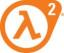# How To Graph This(x^2+y^2+z^2=4)

2 replies to this topic

### #1arash_class

arash_class

Newbie

•• Members
•• 11 posts

Posted 04 June 2007 - 03:51 PM

how to graph this(x^2+y^2+z^2=4) in classpad

### #2The_AFX_Master

The_AFX_Master

Casio Overlord

•• [Legends]
•• 519 posts
• Gender:Male
• Location:Black Mesa Research Facility (sector C)
• Interests:BASIC +FORTRAN 90+ C++.....and HALF LIFE

• Calculators:
Casio Algebra FX 2.0 Plus, Casio fx 570 ms, Classpad 300, And a crowbar

Posted 04 June 2007 - 09:32 PM

Parse Z as a function of X,Y as:

z=+- sqrt(4-x^2-y^2) will give you the upper or the lower part of the graph (+ or -) that's a disadvantage

also you can graph the whole thing with parametric expressions (OS3), but i didnt give it a try, someone else?. or you can see the OS 3.00 users guide, somewhere on the forum,.

### #3Gaumerie

Gaumerie

Newbie

•• Members
•• 28 posts

Posted 05 June 2007 - 06:53 AM

x(s,t) = 4 Cos(s) Sin(t)
y(s,t) = 4 Sin(s) Sin(t)
z(s,t) = 4 Cos(t)

#### 0 user(s) are reading this topic

0 members, 0 guests, 0 anonymous users